# Flashcards for NEET Chemistry - Solutions

Flashcards for NEET Chemistry are designed to boost your NEET preparation. Find below flashcards for the chapter “Solutions”. These flashcards are prepared as per the NEET syllabus. These are helpful for aspirants of NEET and other exams, during last-minute revision. It covers all the important points that are frequently asked in the exam. Check BYJU’S, for the full set of Flashcards and Study material for NEET Chemistry.

 Name of the NEET Sub-section Topic Flashcards Helpful for Chemistry Solutions NEET Exams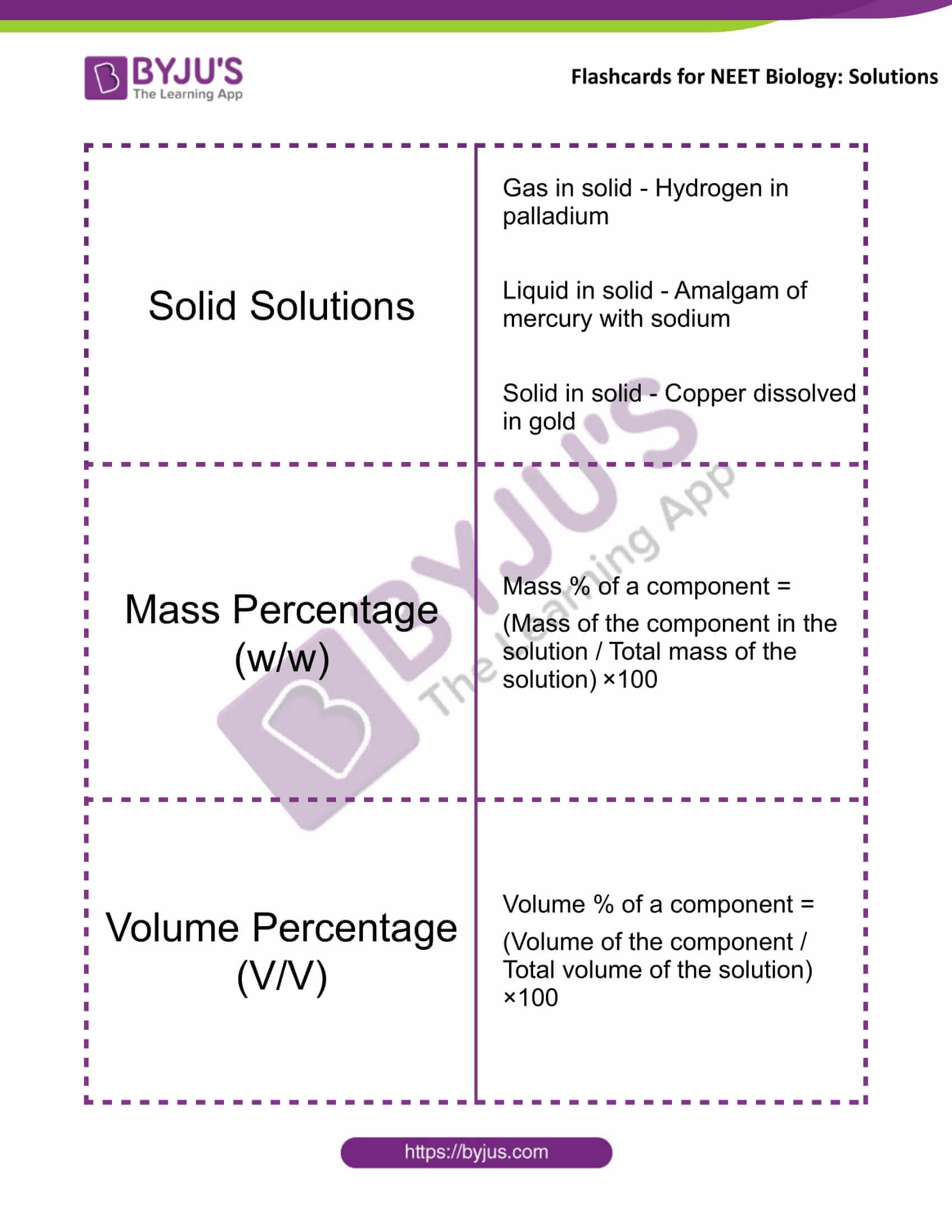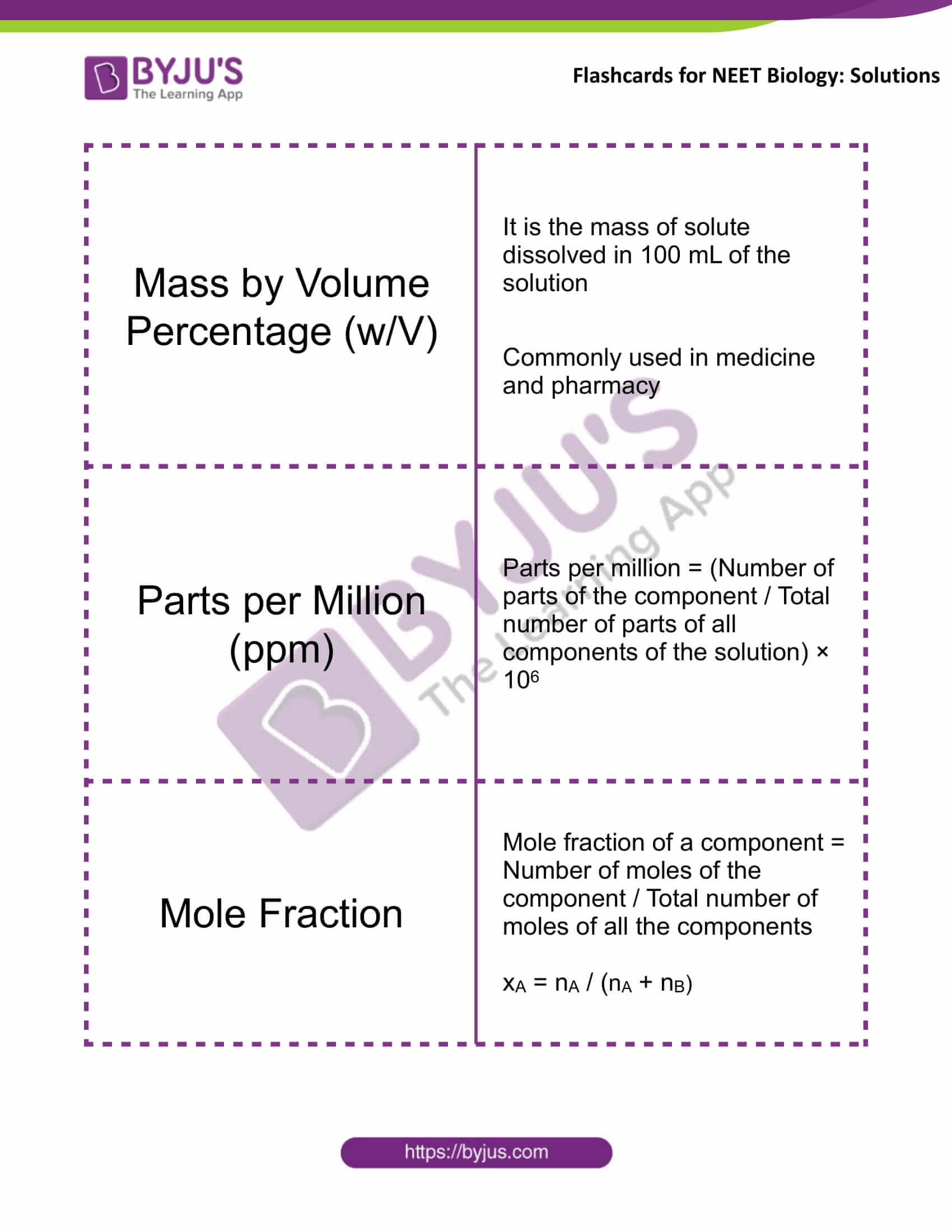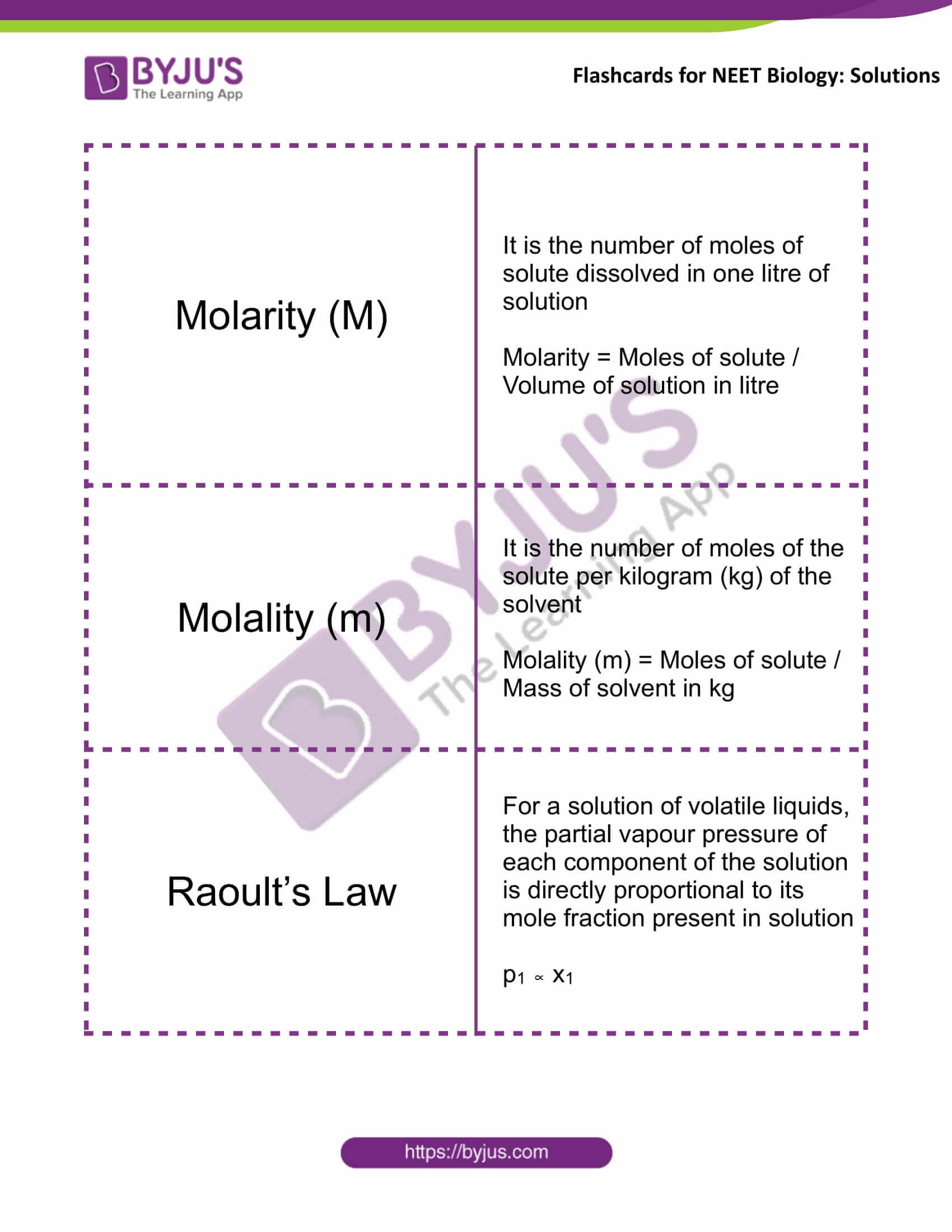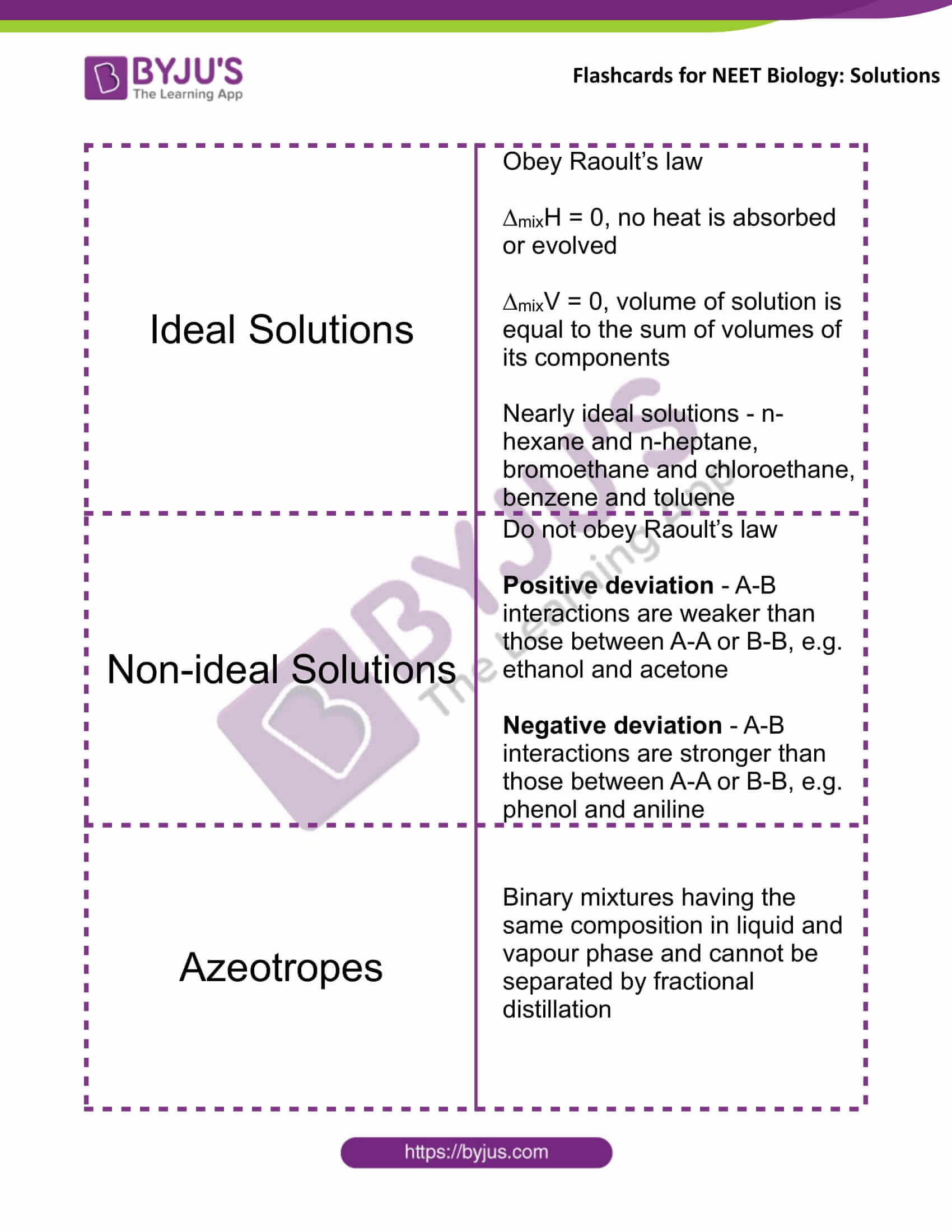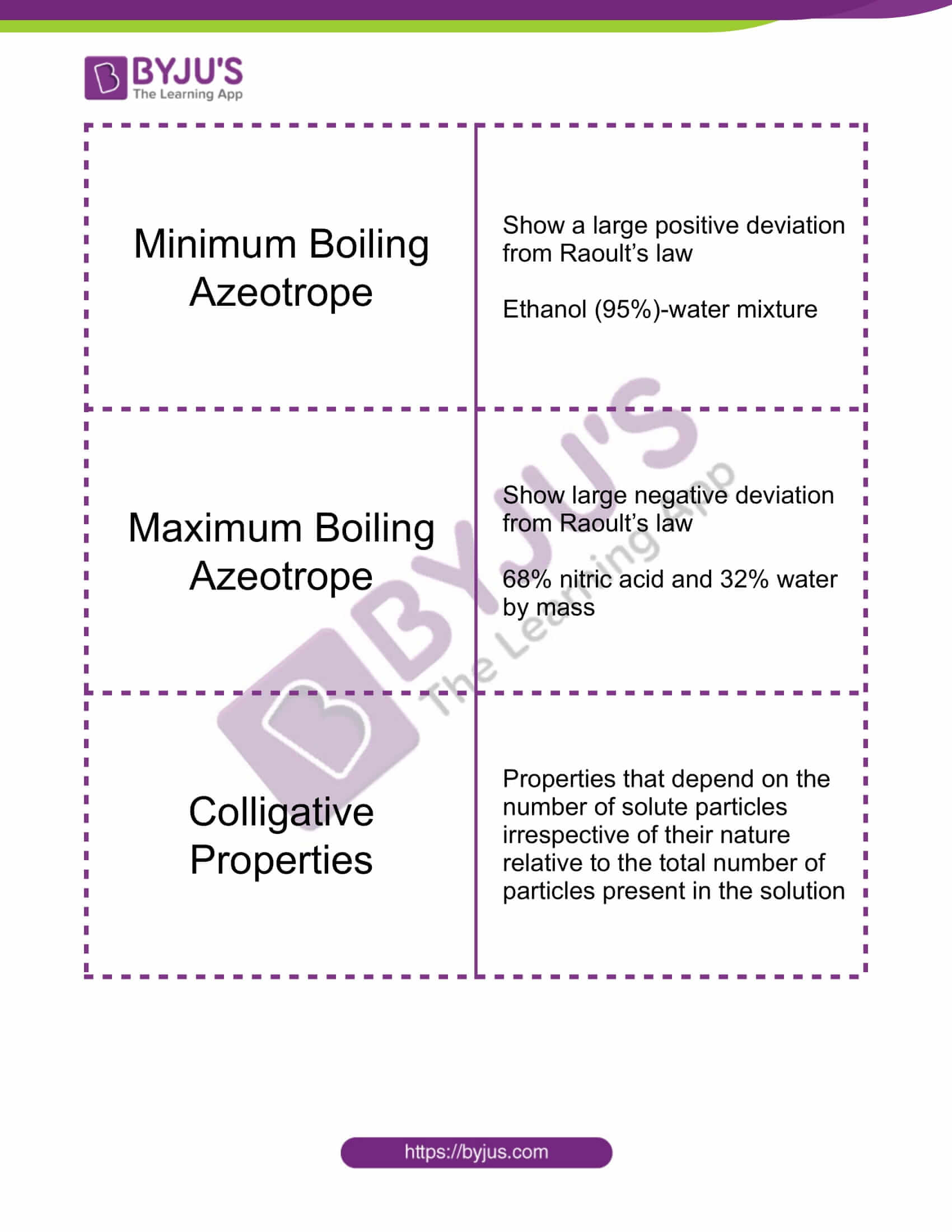Solutions Solid Solutions Gas in solid – Hydrogen in palladium Liquid in solid – Amalgam of mercury with sodium Solid in solid – Copper dissolved in gold Mass Percentage (w/w) Mass % of a component = (Mass of the component in the solution / Total mass of the solution) ×100 Volume Percentage (V/V) Volume % of a component = (Volume of the component / Total volume of the solution) ×100 Mass by Volume Percentage (w/V) It is the mass of solute dissolved in 100 mL of the solution Commonly used in medicine and pharmacy Parts per Million (ppm) Parts per million = (Number of parts of the component / Total number of parts of all components of the solution) × 106 Mole Fraction Mole fraction of a component = Number of moles of the component / Total number of moles of all the components xA = nA / (nA + nB) Molarity (M) It is the number of moles of solute dissolved in one litre of solution Molarity = Moles of solute / Volume of solution in litre Molality (m) It is the number of moles of the solute per kilogram (kg) of the solvent Molality (m) = Moles of solute / Mass of solvent in kg Raoult’s Law For a solution of volatile liquids, the partial vapour pressure of each component of the solution is directly proportional to its mole fraction present in solution p1 ∝ x1 Ideal Solutions Obey Raoult’s law ∆mixH = 0, no heat is absorbed or evolved ∆mixV = 0, the volume of solution is equal to the sum of volumes of its components Nearly ideal solutions – n-hexane and n-heptane, bromoethane and chloroethane, benzene and toluene Non-ideal Solutions Do not obey Raoult’s law Positive deviation – A-B interactions are weaker than those between A-A or B-B, e.g. ethanol and acetone Negative deviation – A-B interactions are stronger than those between A-A or B-B, e.g. phenol and aniline Azeotropes Binary mixtures having the same composition in liquid and vapour phase and cannot be separated by fractional distillation Minimum Boiling Azeotrope Show a large positive deviation from Raoult’s law Ethanol (95%) – water mixture Maximum Boiling Azeotrope Show large negative deviation from Raoult’s law 68% nitric acid and 32% water by mass Colligative Properties Properties that depend on the number of solute particles irrespective of their nature relative to the total number of particles present in the solution

Get access to the full set of flashcards for NEET Chemistry, only at BYJU’S.

### Recommended Video:

Effect of Change in Concentration | CHEMISTRY | NEET | Concept of the Day